# RD Sharma Solutions Class 12 Algebra Of Vectors Exercise 23.8

RD Sharma Solutions for Class 12 Maths Chapter 23 Algebra of Vectors Exercise 23.8 are given here for students to apprehend the important concepts instantly. Referring to these solutions from time to time will help students score high marks in their board exams. Students can now practise the RD Sharma Class 12 Solutions for this exercise from the PDF attached from the download link given below. Further, all these solutions are in accordance with the latest CBSE guidelines.

## Download PDF of Rd Sharma Solution for Class 12 Maths Chapter 23 Exercise 8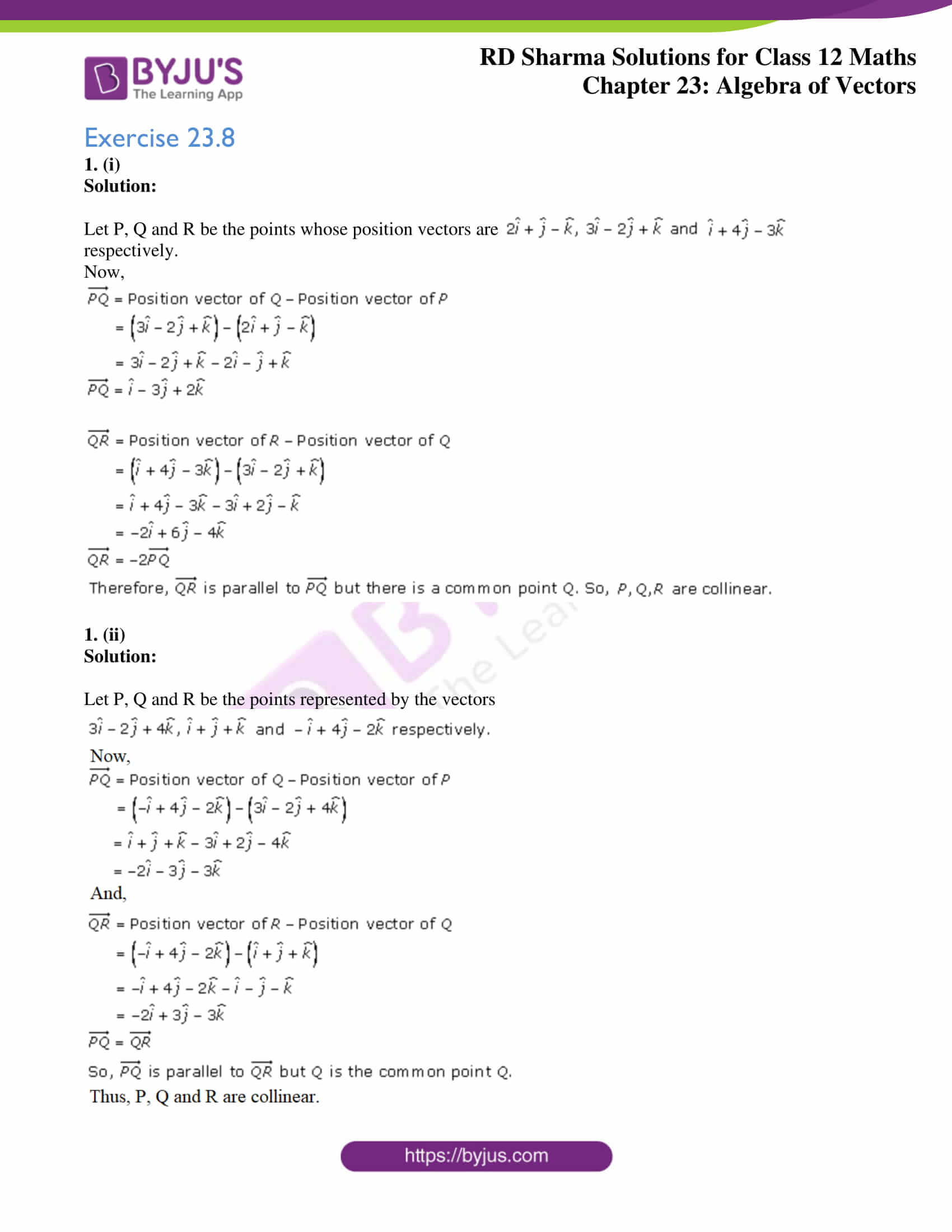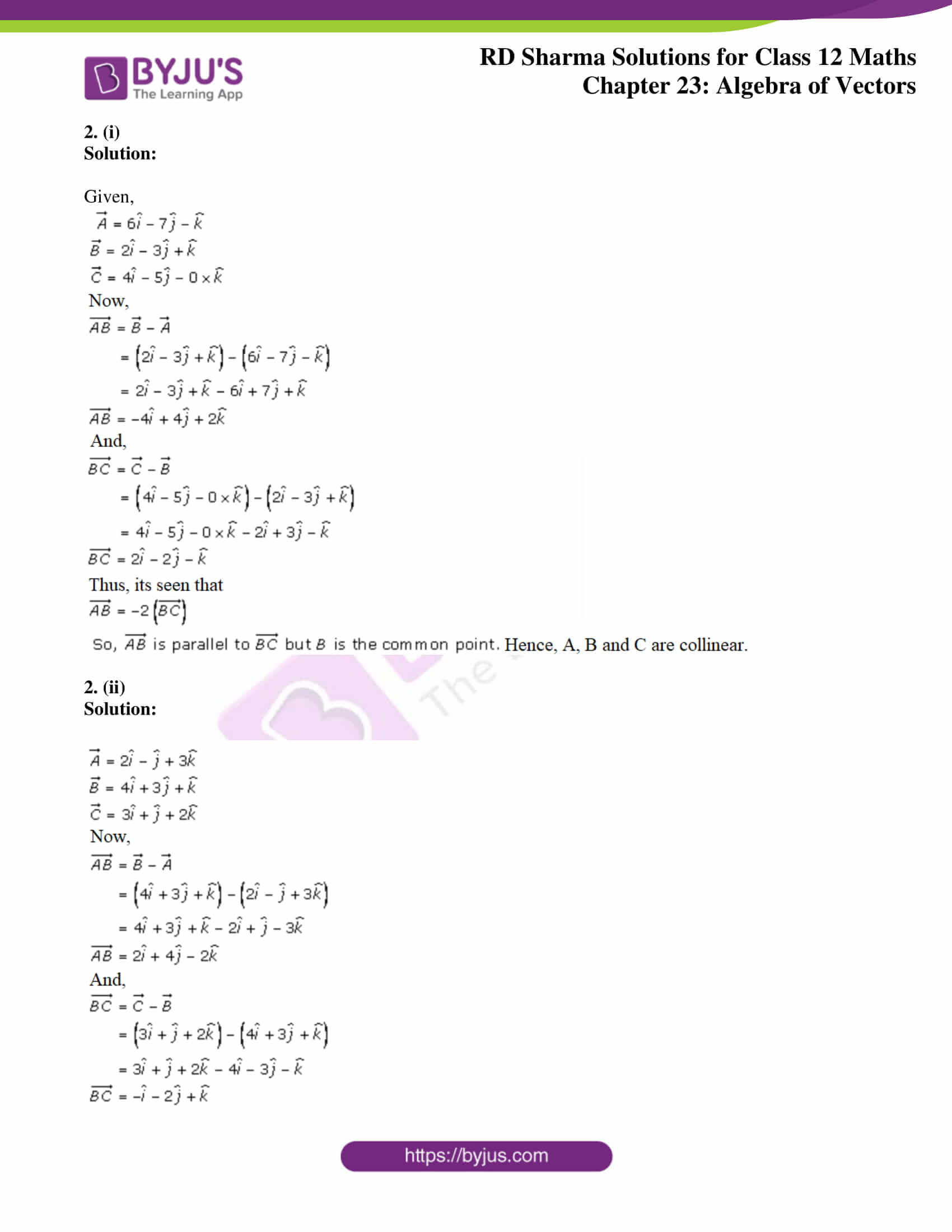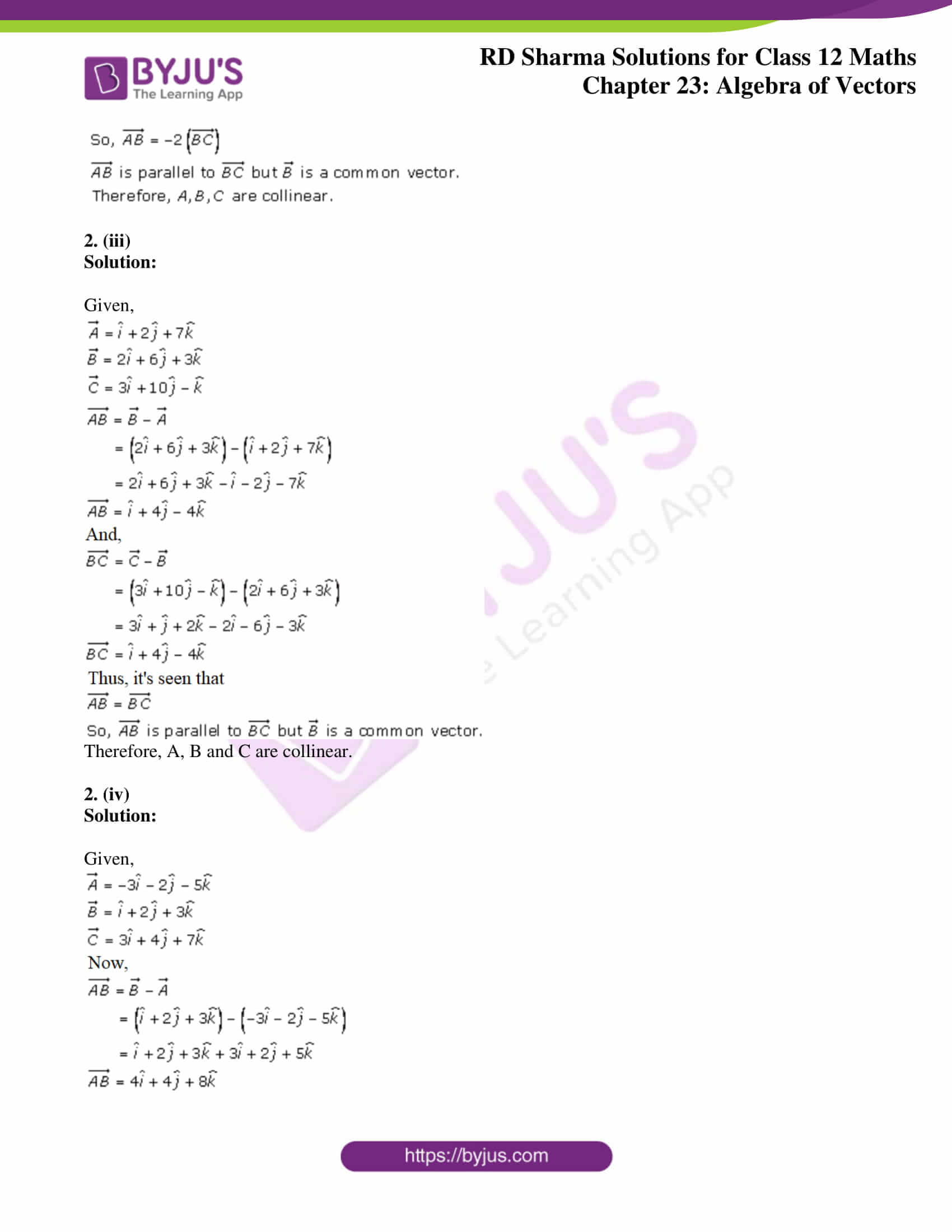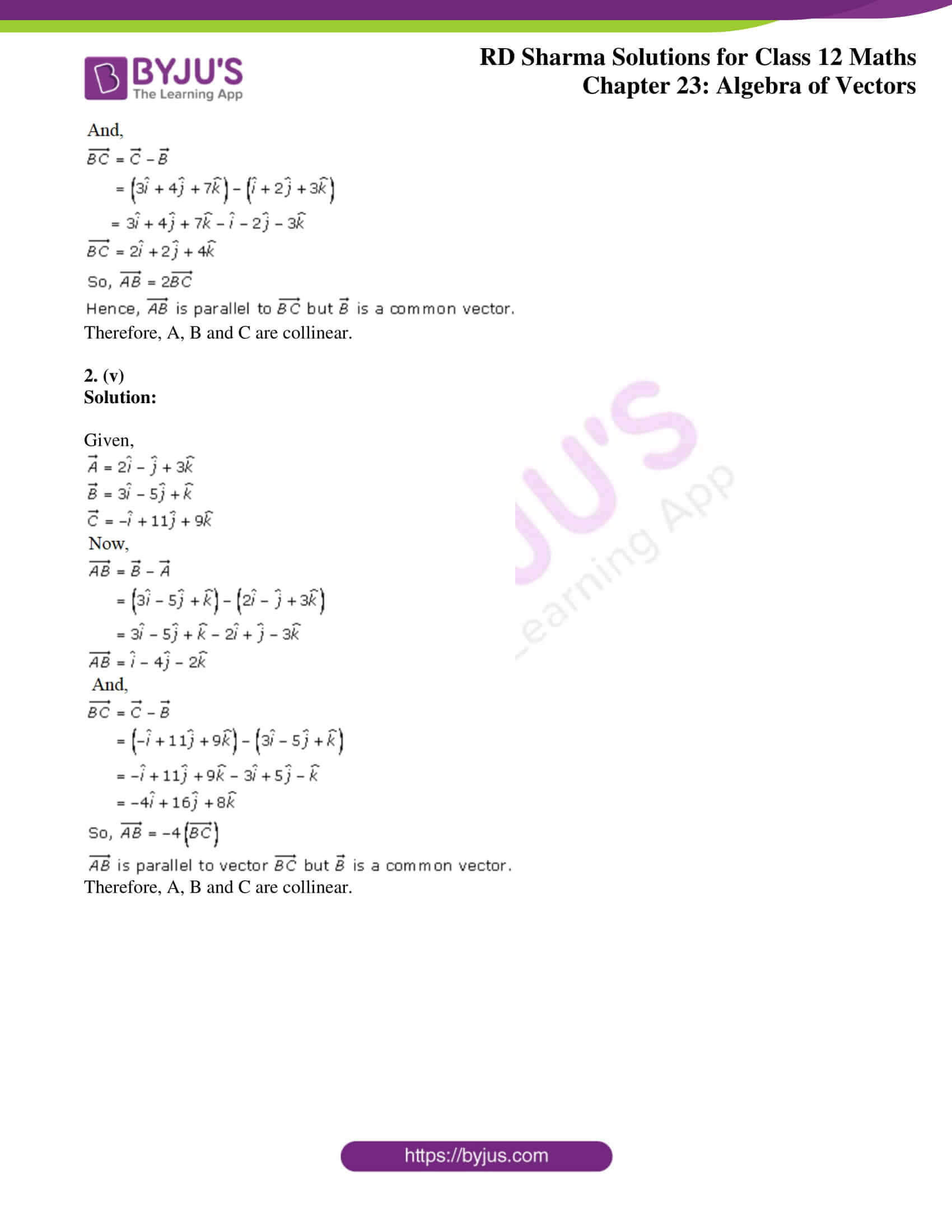### Access Answers for Rd Sharma Solution Class 12 Maths Chapter 23 Exercise 8

Exercise 23.8

1. (i)

Solution: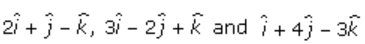Let P, Q and R be the points whose position vectors are

respectively.

Now,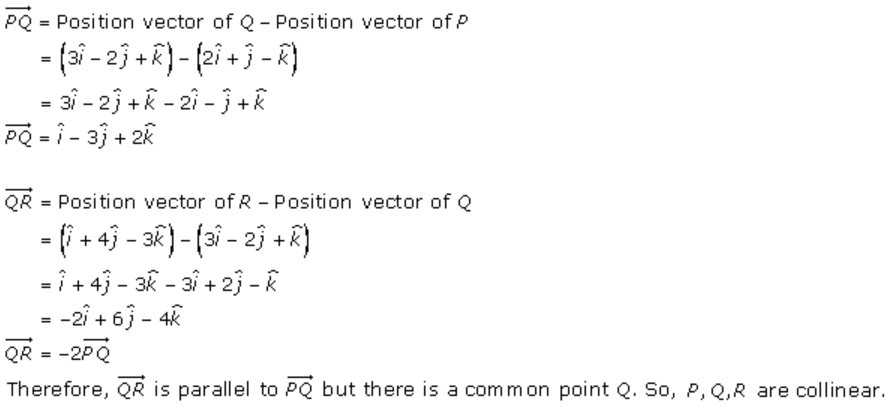1. (ii)

Solution:

Let P, Q and R be the points represented by the vectors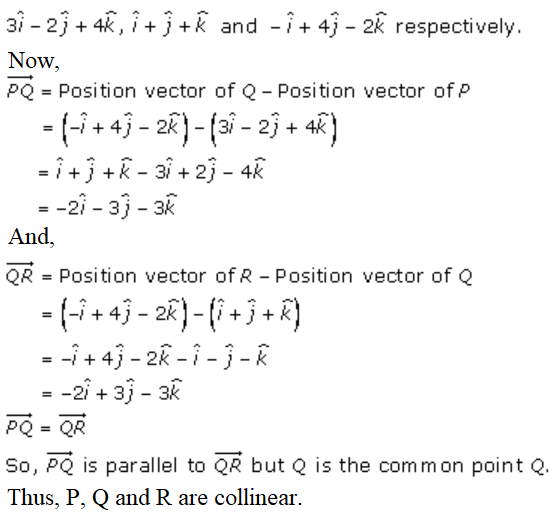2. (i)

Solution:

Given,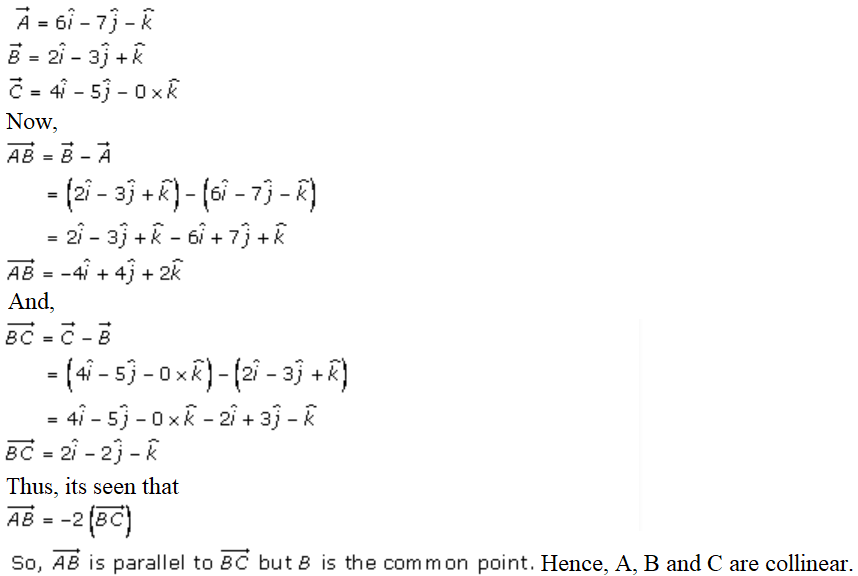2. (ii)

Solution: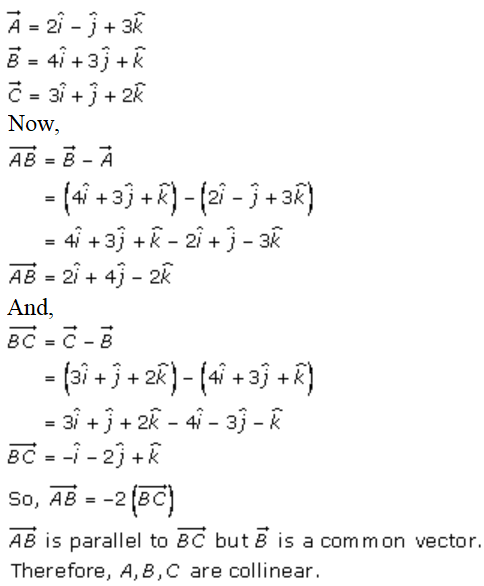2. (iii)

Solution:

Given,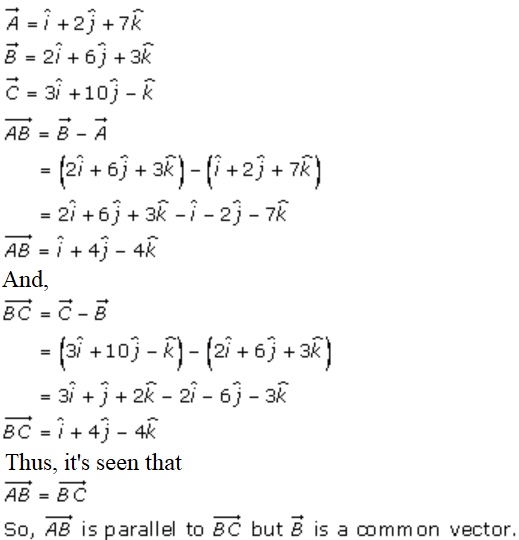Therefore, A, B and C are collinear.

2. (iv)

Solution:

Given,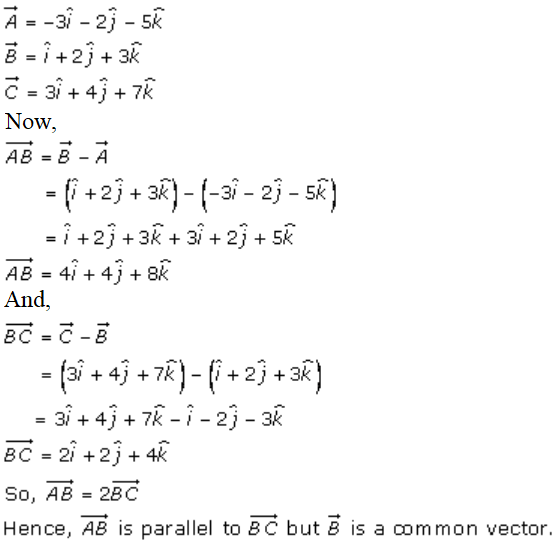Therefore, A, B and C are collinear.

2. (v)

Solution:

Given,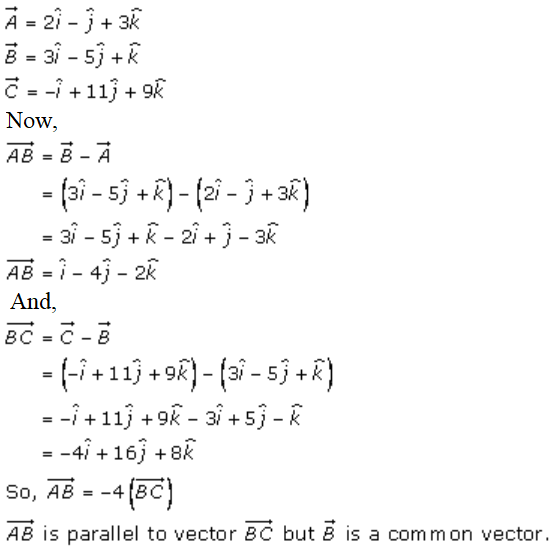Therefore, A, B and C are collinear.